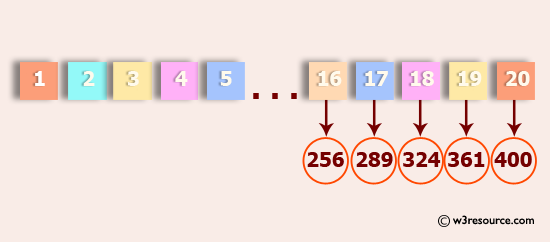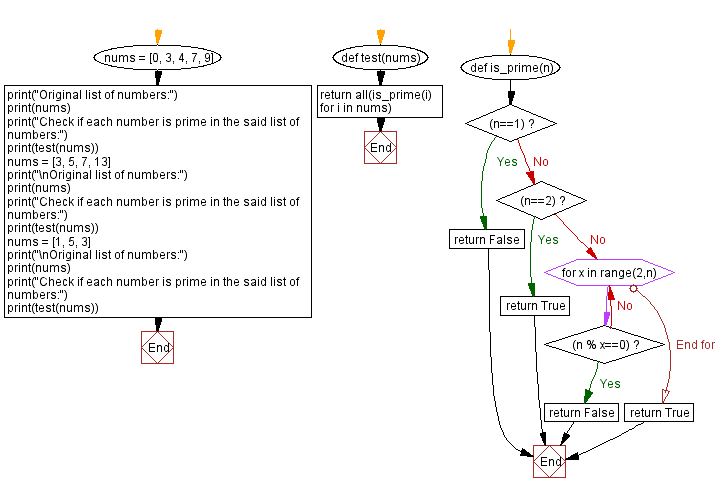﻿ Python: Generate and print a list except for the first 5 elements, where the values are square of numbers between two numbers - w3resource# Python: Generate and print a list except for the first 5 elements, where the values are square of numbers between two numbers

## Python List: Exercise - 17 with Solution

Write a Python program to generate and print a list except for the first 5 elements, where the values are square of numbers between 1 and 30 (both included).Sample Solution:-

Python Code:

``````def printValues():
l = list()
for i in range(1,31):
l.append(i**2)
print(l[5:])
printValues()
```
```

Sample Output:

```[36, 49, 64, 81, 100, 121, 144, 169, 196, 225, 256, 289, 324, 361, 400, 441, 484, 529, 576, 625, 676, 729, 784, 841, 900]
```

Flowchart:## Visualize Python code execution:

The following tool visualize what the computer is doing step-by-step as it executes the said program:

Python Code Editor:

Have another way to solve this solution? Contribute your code (and comments) through Disqus.

What is the difficulty level of this exercise?

Test your Python skills with w3resource's quiz

﻿

## Python: Tips of the Day

Floor Division:

When we speak of division we normally mean (/) float division operator, this will give a precise result in float format with decimals.

For a rounded integer result there is (//) floor division operator in Python. Floor division will only give integer results that are round numbers.

```print(1000 // 300)
print(1000 / 300)```

Output:

```3
3.3333333333333335```